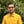Trusted answers to developer questions
Trusted Answers to Developer Questions

Related Tags

swift
cosh
math
communitycreator

# What is the cosh() function in Swift?Harris Amjad

Grokking Modern System Design Interview for Engineers & Managers

Ace your System Design Interview and take your career to the next level. Learn to handle the design of applications like Netflix, Quora, Facebook, Uber, and many more in a 45-min interview. Learn the RESHADED framework for architecting web-scale applications by determining requirements, constraints, and assumptions before diving into a step-by-step design process.

### Overview

The cosh() function returns the hyperbolic cosine of a number.

The figure below shows the mathematical representation of the cosh() function:

Mathematical representation of hyperbolic cosine function

Note: We need to import Foundation in our code to use the cosh() function. We can import it by using import Foundation.

### Syntax

cosh(num)


### Parameter

This function requires a number that represents an angle in radians as a parameter.

To convert degrees to radians, we use the following formula:

radians = degrees * ( PI / 180.0 )


### Return value

The cosh() function returns the hyperbolic cosine of a number sent as a parameter.

### Example

The code below shows how to use the cosh() function in swift:

import Swiftimport Foundation//positive number in radiansprint("The value of cosh(2.3) :", cosh(2.3));// negative number in radiansprint("The value of cosh(-2.3) :", cosh(-2.3));//converting the degrees angle into radians and then applying cosh()// degrees = 180.0// PI = 3.14159265print("The value of cosh(180.0 * (PI / 180.0)) :", cosh(180.0 * (Double.pi / 180.0)));
Example of cosh() function in Swift

### Code explanation

• Line 2: We add the Foundation header required for the cosh() function.
• Line 5: We calculate the hyperbolic cosine of the positive number in radians using cosh().
• Line 8: We calculate the hyperbolic cosine of the negative number in radians using cosh().
• Line 13: We convert the angle in degrees to radians and then calculate its hyperbolic cosine using cosh().

RELATED TAGS

swift
cosh
math
communitycreator

CONTRIBUTORHarris Amjad

Grokking Modern System Design Interview for Engineers & Managers

Ace your System Design Interview and take your career to the next level. Learn to handle the design of applications like Netflix, Quora, Facebook, Uber, and many more in a 45-min interview. Learn the RESHADED framework for architecting web-scale applications by determining requirements, constraints, and assumptions before diving into a step-by-step design process.

Keep Exploring

Learn in-demand tech skills in half the time